# OECD Purchasing power parities (PPP) data analysis 6 - Time-Series Analysis using R. Static Time Series ModelPhoto by Sora Sagano on Unsplash

This post is following of the above post.

In this post, I will do time-series analysis. I use JPN data only.

Firstly, I make JPN only dataframe.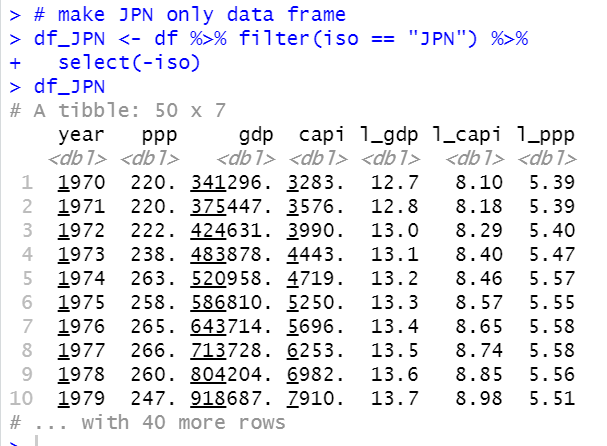Then, let's see statistical summary of df_JPN.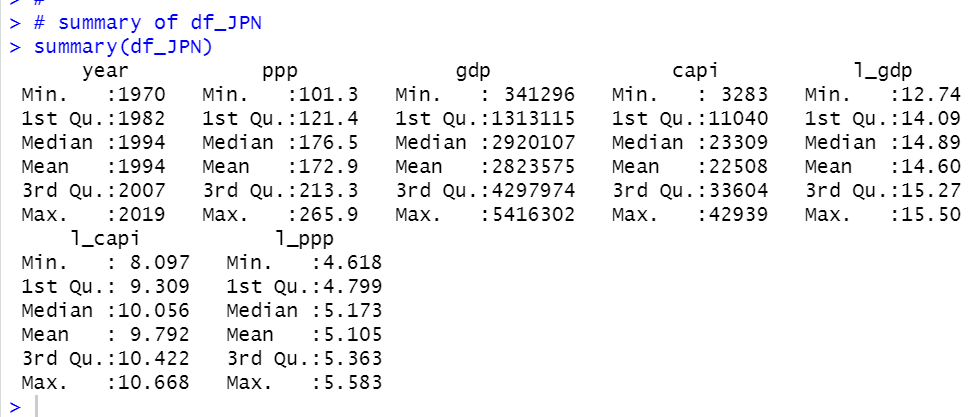summary() function does not show standard deviations and CV (Coefficient of Variation).

So, let's calculate it.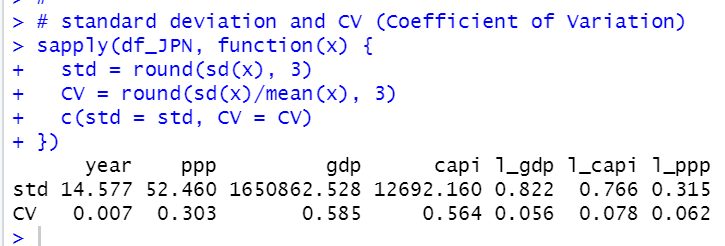gdp is the most variable data and l_ppp is the least variable data excluding year.

Let's see correlation matrix.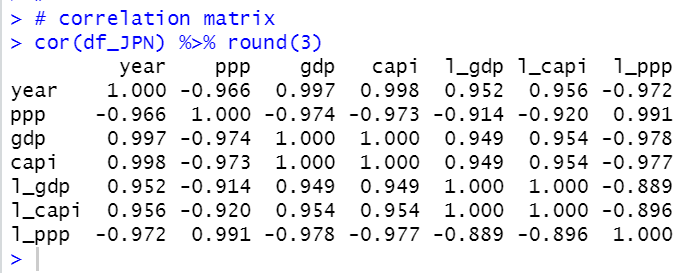I see each variables are strongly correlated each other.

For time-series analysis, it is convinenent to use ts object, so I will make ts object from df_JPN and name it as ts_JPN.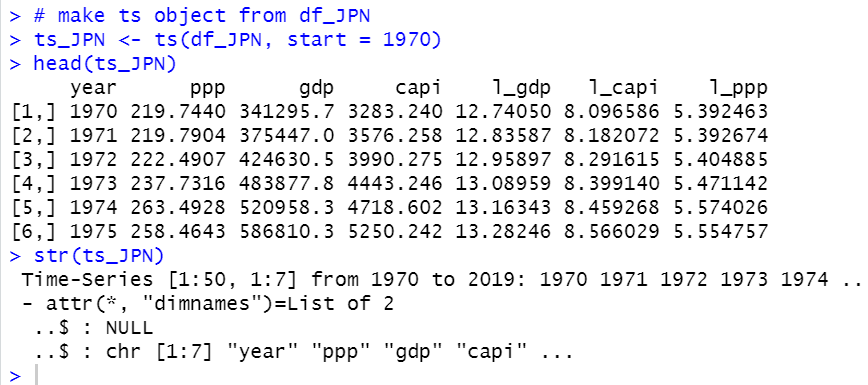plot() function can make a line chart with ts object like below.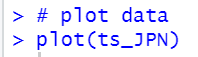I will make a static time-serires model.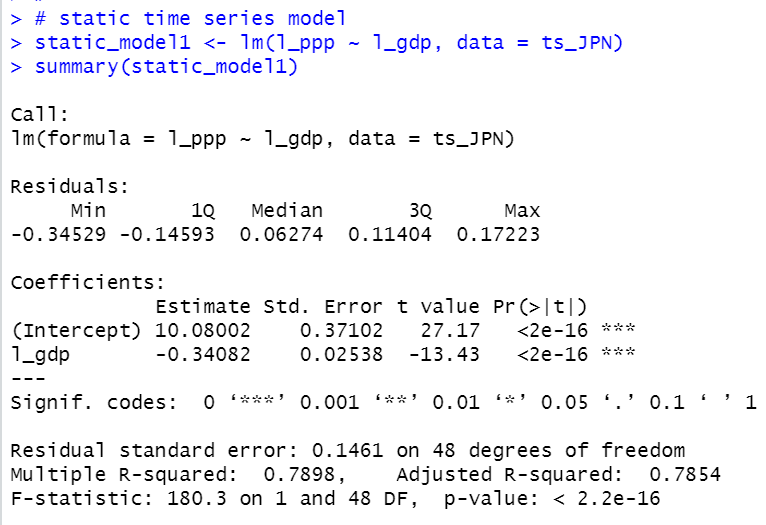Above model means

l_ppp = -10.08 -0.34*l_gdp + u

gdp inclease by 1%, ppp will decrease by 0.34%.

In time-series analysis, we have to check if there is serieal correlation.

I make an object for residuals and plot it.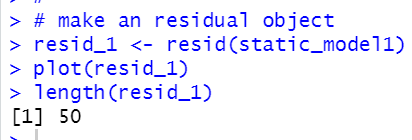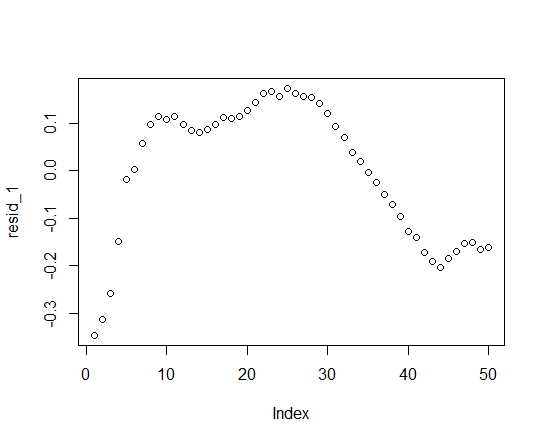I see there are 50 residual values and above plot shoes there seems serial correlation.

Let's see if there is residual(t) and residual(t-1) correlation. Firstly, let's see a scatter plot.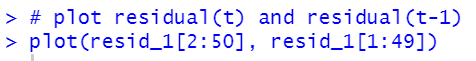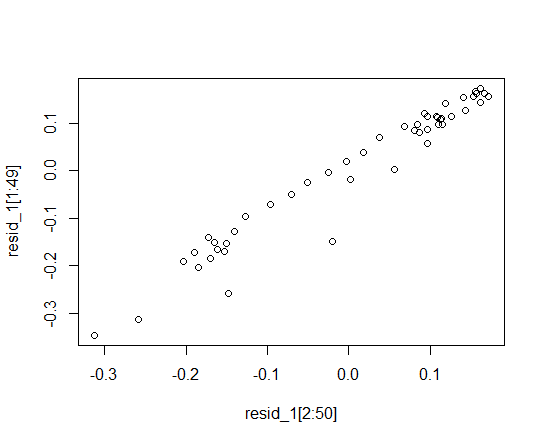We see there is strong positive correlation between residual(t) and residual(t-1).

Let's do linear regression and see t-statistics.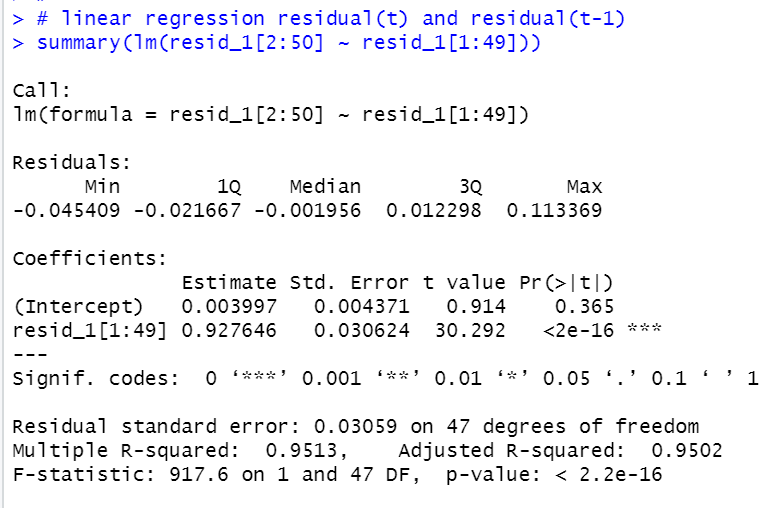t value is 30.292 and p-value is almost 0. So, we can say there is serial correlation in

l_ppp  = 10.8 - 0.34*l_gdp + u

That's it. Than you!

To read from the 1st post,